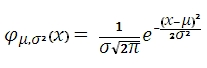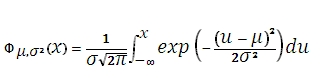# SQL Server NORM.DIST function

Updated: 9 August 2010

Use NORMDIST to calculate the normal distribution for the specified mean and standard deviation. The formula for the probability density function (pdf) is:The formula for the cumulative distribution function (cdf) is:Syntax
SELECT [wctStatistics].[wct].[NORMDIST] (
<@X, float,>
,<@Mean, float,>
,<@Standard_dev, float,>
,<@Cumulative, bit,>)
Arguments
@X
is the value at which to evaluate the function. @X is an expression of type float or of a type that can be implicitly converted to float
@Mean
is the arithmetic mean of the distribution. @Mean is an expression of type float or of a type that can be implicitly converted to float
@Standard_dev
is the standard deviation of the distribution. @Standard_dev is an expression of type float or of a type that can be implicitly converted to float
@Cumulative
is a logical value that determines if the probability density function (False, 0) or the cumulative distribution function (True, 1) is being calculated.
Return Types
float
Remarks
·         If @Standard_dev ≤ 0, NORMDIST returns an error
·         If @Mean = 0 and @Standard_dev = 1 and @Cumulative = 'False', NORMDIST = NORMSDIST(@X)
Examples

Select
wct.NORMDIST(-4.6159,10,5,'False')

This produces the following result

----------------------
0.00111280980994974

(1 row(s) affected)

Select
wct.NORMDIST(-4.6159,10,5,'True')

This produces the following result

----------------------
0.00173238078965338

(1 row(s) affected)Copyright 2008-2023 Westclintech LLC         Privacy Policy        Terms of Service